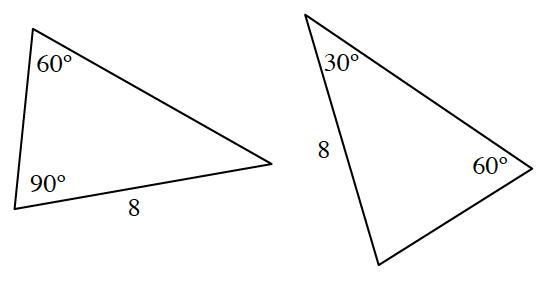### Home > INT1 > Chapter 10 > Lesson 10.1.3 > Problem10-48

10-48.

Are the triangles congruent? If so, explain how you know with a flowchart (you may need to label the vertices), and then indicate a sequence of transformations that maps one triangle onto the other. If not, explain why not. The triangles are not drawn to scale.Review the Math Notes box in section 7.1.7

Calculate the missing angle for each triangle.
Consider which triangle congruence condition could be met by these two triangles.